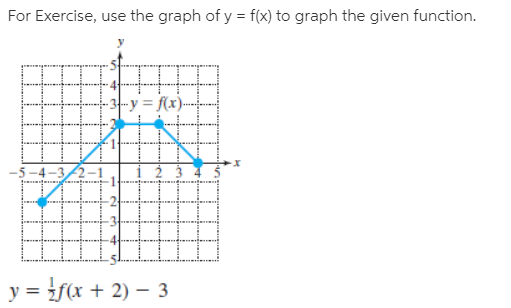# For Exercise, use the graph of y = f(x) to graph the given function. -y = f(x)- -5-4-3 y = f(x + 2) – 3

Questionhelp_outlineImage TranscriptioncloseFor Exercise, use the graph of y = f(x) to graph the given function. -y = f(x)- -5-4-3 y = f(x + 2) – 3 fullscreen